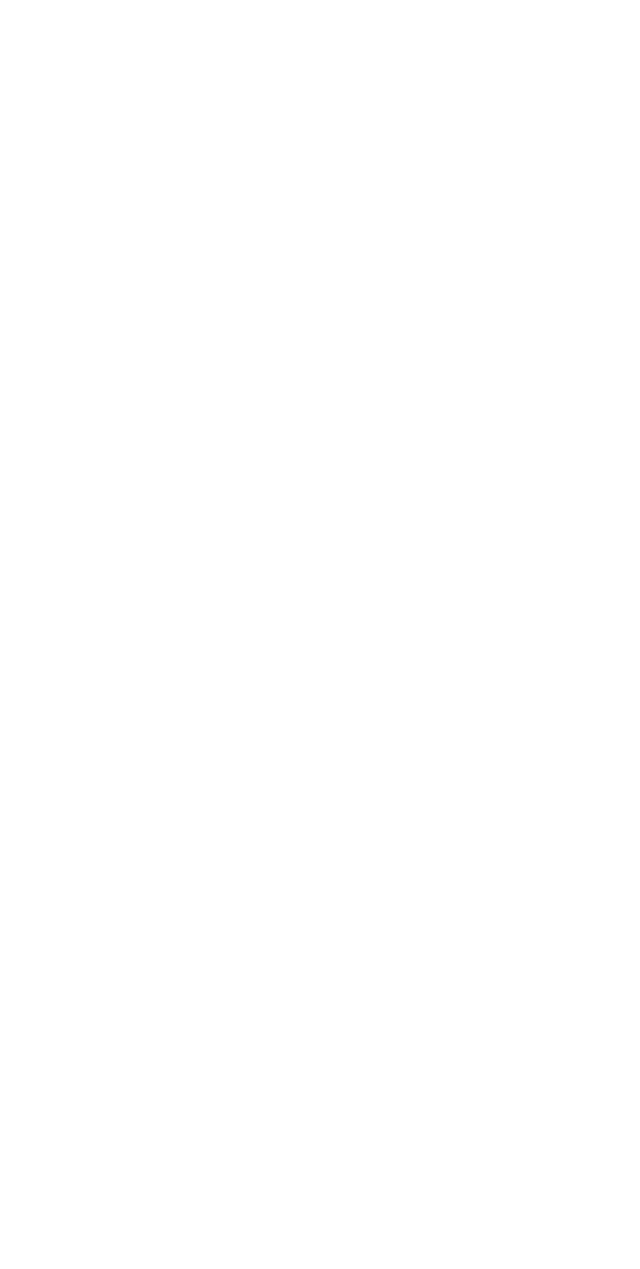# Application of derivatives in Economics and Commerce

APPLICATION OF CALCULUS IN COMMERCE AND ECONOMICS

We have learnt in calculus that when ‘y’ is function of ‘x’, the derivative of y with respect to x i.e. (dy/dx) measures the rate of change of y with respect to x. In Economics and commerce we come across many such variables where one variable is a function of the another variable. For example, the quantity demanded can be said to be a function of price “x” . Supply and price or cost and quantity demanded are some many other such variables. Calculus helps us in finding the rate at which one quantity changes with respect to the other. Marginal analysis in Economics and Commerce is the direct application of differential calculus. In this context, differential calculus also helps solve problems of finding maximum profit or minimum cost etc., while integral calculus is used to find the cost function when the marginal cost is given and to find total revenue when marginal revenue is given.

After the use of this article, you will be able to: Define Total Cost, Variable Cost, Fixed Cost, Demand Function and Total Revenue Function.

1. Cost Function C(x)
The total cost C of producing and marketing x units of a product depends upon the number of units (x). So the function relating C and x is called Cost-function and is written as C = C (x).

The total cost of producing x units of the product consists of two parts
i. Fixed Cost
ii. Variable Cost i.e. C (x) = F + V (x)

Fixed Cost : The fixed cost consists of all types of costs which do not change with the level of production. For example, the rent of the premises, the insurance, taxes, etc.
Variable Cost : The variable cost is the sum of all costs that are dependent on the level of production. For example, the cost of material, labour cost, cost of packaging, etc.

2. Demand Function
An equation that relates price per unit and quantity demanded at that price is called a demand function.
If ‘p’ is the price per unit of a certain product and x is the number of units demanded, then we can write the demand function as x = f(p)
or p = g (x) i.e., price (p) expressed as a function of x.

3. Revenue function
If x is the number of units of certain product sold at a rate of Rs. ‘p’ per unit, then the amount derived from the sale of x units of a product is the total revenue. Thus, if R represents the total revenue from x units of the product at the rate of Rs. ‘p’ per unit then
R= p.x is the total revenue Thus, the Revenue function R (x) = p.x. = x .p (x)

4. Profit Function
The profit is calculated by subtracting the total cost from the total revenue obtained by selling x units of a product. Thus, if P (x) is the profit function, then
P(x) = R(x) − C(x)

~*~